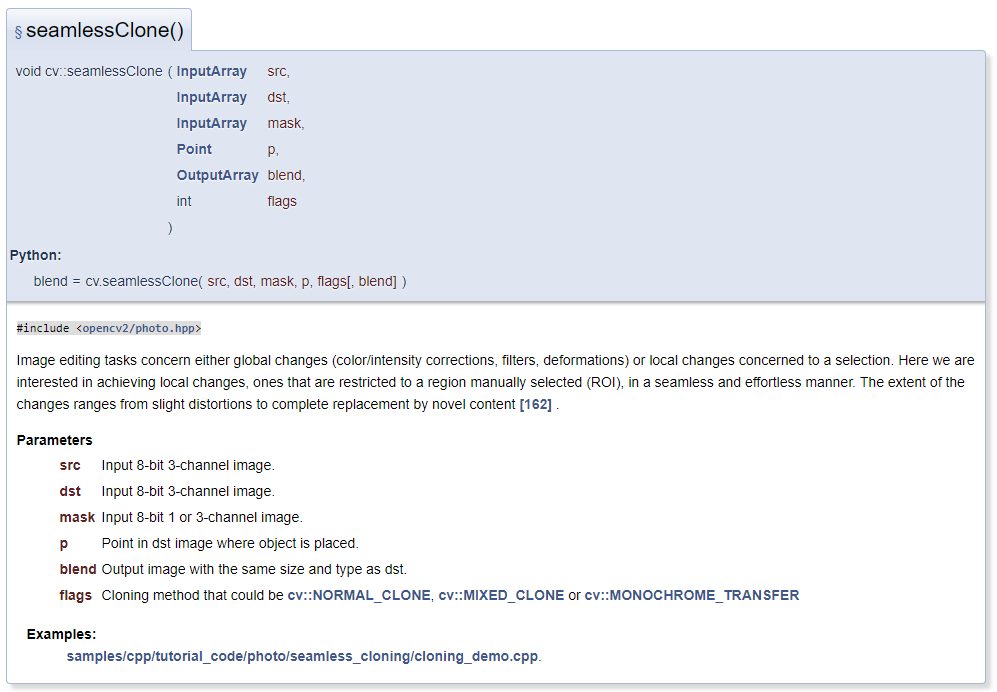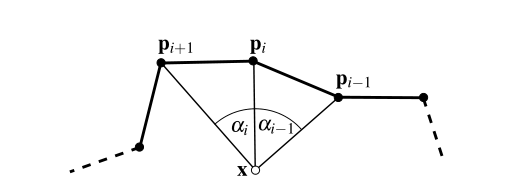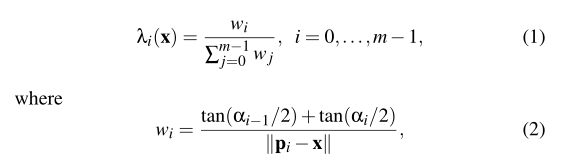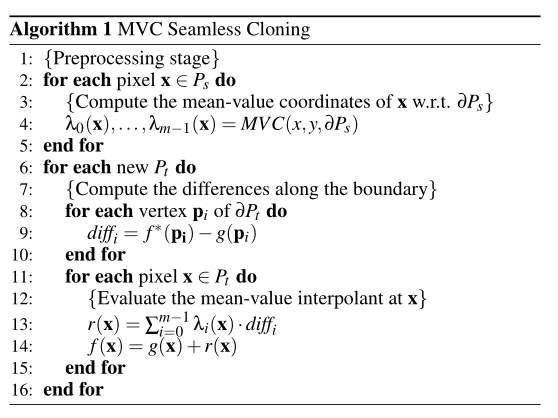# 2. 实现

## 2.1. 准备《使用QT显示OpenCV读取的图片》
《使用QT绘制一个多边形》

## 2.2. 核心

### 2.2.1. 均值坐标（Mean-Value Coordinates）### 2.2.3. 核心实现1. 假设ROI区域内有n个点，其边界由m个点组成。
2. 那么可以求每个点的MVC(均值坐标)，每个点有m个坐标值，一共有n个点，MVC就是就是一个n*m的矩阵。
3. 求ROI区域边界的像素差diff，显然其是一个m*1的矩阵。
4. 那么新图像ROI区域的插值为：r = MVC * diff，矩阵乘法后r为n*1矩阵。
5. 将插值r与原图像g矩阵相加：f = g + r，替换目标图像相应位置的值。

    QTime startTime = QTime::currentTime();

//Step1:找到边界上所有的像素点
vector<Vector2d> ROIBoundPointList;
CalBoundPoint(ROIBoundPointList);

//Step2:计算范围内每个点的 mean-value coordinates
size_t srcImgBufNum = static_cast<size_t>(srcImg.cols) * static_cast<size_t>(srcImg.rows);
vector<vector<double>> MVC(srcImgBufNum);
for(size_t i = 0; i < srcImgBufNum; i++)
{
MVC[i].resize(ROIBoundPointList.size()-1, 0);
}
vector<bool> clipMap(srcImgBufNum, true);           //标识范围内的点

cout<<"开始计算 mean-value coordinates..." << endl;
#pragma omp parallel for        //开启OpenMP并行加速
for (int ri = 0; ri < srcImg.rows; ++ri)
{
for (int ci = 0; ci < srcImg.cols; ++ci)
{
//点是否在多边形内
size_t m = static_cast<size_t>(srcImg.cols) * ri + ci;
if(!Point_In_Polygon_2D(ci, ri, ROIBoundPointList))
{
clipMap[m] = false;
continue;
}

//逐点计算MVC
Vector2d P(ci, ri);
vector<double> alphaAngle(ROIBoundPointList.size());
for(size_t pi = 1; pi < ROIBoundPointList.size(); pi++)
{
alphaAngle[pi] = threePointCalAngle(ROIBoundPointList[pi-1], P, ROIBoundPointList[pi]);
}
alphaAngle = alphaAngle[ROIBoundPointList.size()-1];

for(size_t pi = 1; pi < ROIBoundPointList.size(); pi++)
{
double w_a = tan(alphaAngle[pi-1]/2) + tan(alphaAngle[pi]/2);
double w_b = (ROIBoundPointList[pi-1] - P).Mod();
MVC[m][pi-1] = w_a / w_b;
if(_isnan(MVC[m][pi-1])==1)
{
MVC[m][pi-1] = 0;
}
}

double sum = 0;
for(size_t pi = 0; pi < MVC[m].size(); pi++)
{
sum = sum + MVC[m][pi];
}

for(size_t pi = 0; pi < MVC[m].size(); pi++)
{
MVC[m][pi] = MVC[m][pi] / sum;
}
}
}
cout<<"计算完成！" << endl;

//Step3:计算边界的像素插值
vector<int> diff;
for(size_t i = 0; i < ROIBoundPointList.size()-1; i++)
{
size_t l = (size_t) srcImg.cols * ROIBoundPointList[i].y + ROIBoundPointList[i].x;
for(int bi = 0; bi < winBandNum; bi++)
{
size_t m = (size_t) dstImg.cols * winBandNum * (ROIBoundPointList[i].y + posY)+ winBandNum * (ROIBoundPointList[i].x + posX) + bi;
size_t n = (size_t) srcImg.cols * winBandNum * ROIBoundPointList[i].y + winBandNum * ROIBoundPointList[i].x + bi;
int d = (int)(dstImg.data[m]) - (int)(srcImg.data[n]);
diff.push_back(d);
}
clipMap[l] = false;         //在多边形边上的点没法计算MVC
}

//Step4:插值计算
cout<<"开始插值计算..." << endl;
//Mat rMat(srcImg.rows, srcImg.cols, CV_64FC3);
#pragma omp parallel for
for (int ri = 0; ri < srcImg.rows; ++ri)
{
for (int ci = 0; ci < srcImg.cols; ++ci)
{
size_t l = (size_t) srcImg.cols * ri + ci;
if(!clipMap[l])
{
continue;
}

vector<double> r(winBandNum, 0);

for(size_t pi = 0; pi < MVC[l].size(); pi++)
{
for(int bi = 0; bi < winBandNum; bi++)
{
r[bi] = r[bi] + MVC[l][pi] * diff[pi * winBandNum + bi];
}
}

for(int bi = 0; bi < winBandNum; bi++)
{
size_t n = (size_t) srcImg.cols * winBandNum * ri + winBandNum * ci + bi;
size_t m = (size_t) dstImg.cols * winBandNum * (ri + posY)+ winBandNum * (ci + posX) + bi;

dstImg.data[m] = min(max(srcImg.data[n] + r[bi], 0.0), 255.0);
}
}
}
cout<<"插值完成！" << endl;

QTime stopTime = QTime::currentTime();
int elapsed = startTime.msecsTo(stopTime);
cout<<"总结完成用时"<<elapsed<<"毫秒";


### 2.2.4. 实现中的问题

1. ROI边界上的点无法计算MVC值，需要予以剔除，否则ROI边界上会出现一圈白色的点。
2. 用到了OpenMP加速，可以大幅提高性能。如有必要的话，可以通过显卡加速。

# 3. 效果

## 3.1. 使用过程# 4. 参考

posted @ 2020-03-07 12:52  charlee44  阅读(1821)  评论(0编辑  收藏  举报